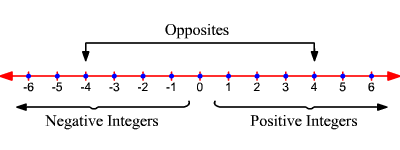# Word Problems Involving Integers

The integers are the set of whole numbers and their opposites . Fractions and decimals are not included in the set of integers.

For example, the numbers $3,7,0,-14,356,-25,\text{\hspace{0.17em}}\text{\hspace{0.17em}}\text{and}\text{\hspace{0.17em}}\text{\hspace{0.17em}}10$ are all integers.

The numbers such as $9.6$ , $\frac{3}{5}$ , and $3\frac{2}{7}$ are not integers.

Integers are used in real world situations. For example, the positive integers are used to denote the height above the sea level, the increase in certain things, and so on. Likewise the negative integers are used to denote the height below the sea level, the decrease in certain things, and so on.

The integers are often represented as equally spaced points on a number line , as shown in the figure.Example:

A hole is drilled in the ground to the depth of $10$ ft and a ball is dropped into it.

What number would you use to represent the position of the ball compared to ground level? Does this number belong to the set of whole numbers? Does it belong to the set of integers?

Usually, ground level is considered as height zero. As you go below this level, the numbers are written as negatives.

The number that represents the position of the ball in feet is $-10$ . This number is an integer, but not a whole number.